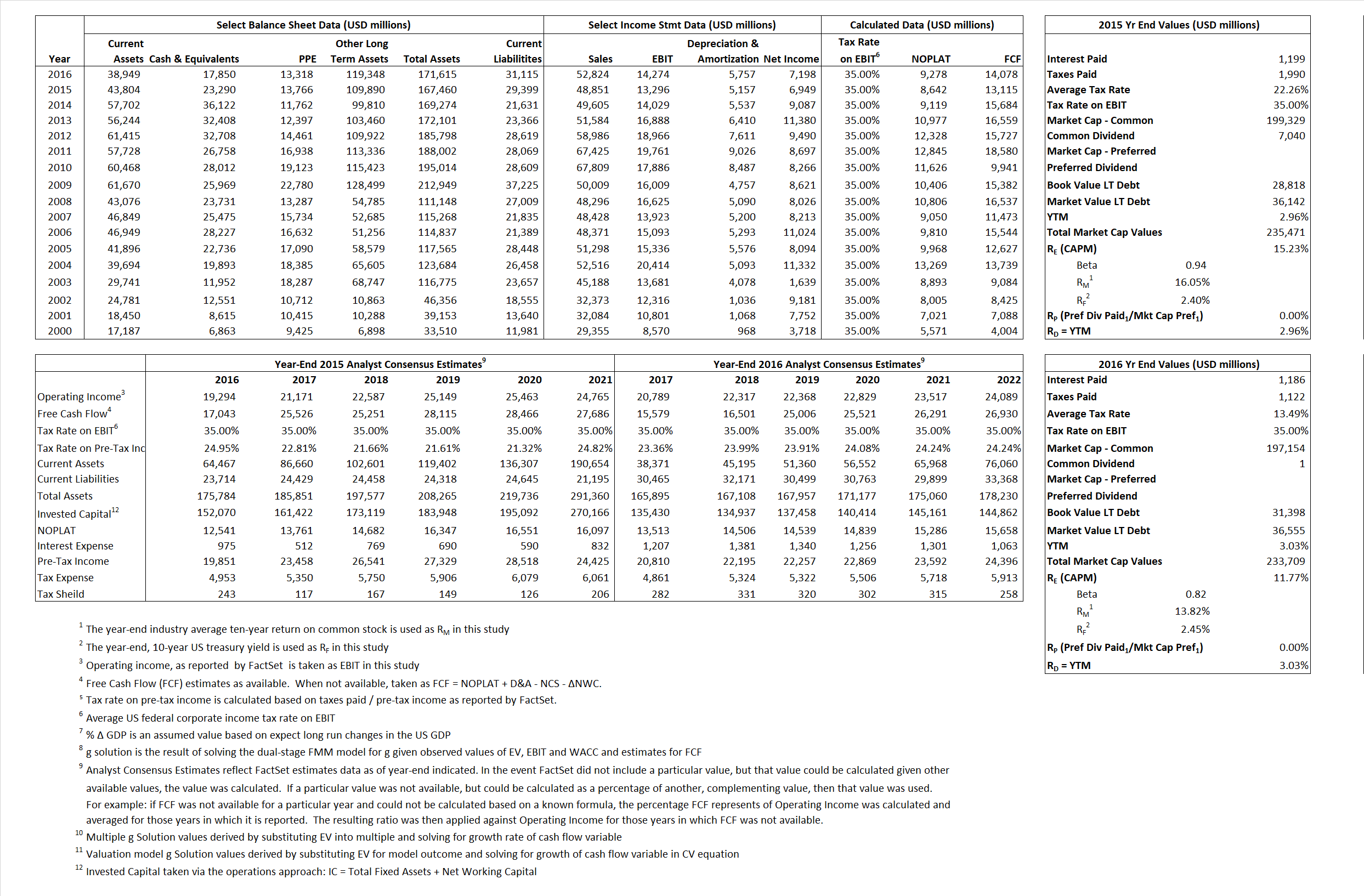# Pfizer Inc

## Analyst Listing

The following analysts provide coverage for the subject firm as of May 2016:

 Broker Analyst Analyst Email BMO Capital Markets Alex Arfaei alex.arfaei@bmo.com Deautche Bank Research Gregg Gilbert gregg.gilbert@db.com SunTrust Robinson Humphrey John T. Boris john.t.boris@suntrust.com Evercore ISI Mark Schoenebaum mark.schoenebaum@evercoreisi.com Piper Jaffray Richard J. Purkiss richard.j.purkiss@pjc.com Leerink Partners Seamus Fernandez seamus.fernandez@leerink.com Atlantic Equities Steve Chesney s.chesney@atlantic-equities.com Cowen & Company Steve Scala steve.scala@cowen.com Bernstein Research Tim Anderson tim.anderson@bernstein.com Credit Suisse Vamil Divan vamil.divan@credit-suisse.com

## Primary Input Data## Derived Input Data

### Equational Form

Net Operating Profit Less Adjusted Taxes NOPLAT 8,642 9,278$NOPLAT\, =\, EBIT\, x\, (1 \,-\, Avg \,\,Tax\,\, Rate\,\, on\,\, EBIT)$
Free Cash Flow FCF 13,115 14,078$FCF\,=NOPLAT\,+\,Non-Cash\,Expenses-\Delta NWC\,-\,NCS$
Tax Shield TS 267 160$TS\,=\,Interest\,\,Paid\,\,x\,\, Avg \,\,Tax\,\,Rate\,\, on\,\, Pre-Tax\,\, Income$
Invested Capital IC 138,061 140,500$IC\,=\,Fixed\,\,Operating\,\,Assets\,\,+\,\,Net\,\, Working\,\, Capital$
Return on Invested Capital ROIC 6.26% 6.60%$ROIC\,=\,\frac { NOPLAT }{ IC }$
Net Investment NetInv (4,425) 8,196$NetInv\,=\,{ {IC}_{1}}-{{IC}_{0}}+Depreciation$
Investment Rate IR -51.20% 88.34%$IR\,=\,\frac {NetInv}{NOPLAT}$
Weighted Average Cost of Capital
WACCMarket  13.25% 10.34%$WACC\,=\,\frac { E }{ V } { R }_{ E }\,+\,\frac { P }{ V } { R }_{ P }\,+\,\frac { D }{ V } { R }_{ D }\left( 1- Avg\,\, Tax\,\,Rate\,\,on\,\,Pre-Tax\,\,Income \right)$
WACCBook   7.79%  6.80%
Enterprise value
EVMarket  212,181 215,859$EV\,=\,Market\,\,Cap\,\,Equity\,+\,\,Long\,\,Term\,\,Debt\,-\,Cash$
EVBook  210,297  210,702
Long-Run Growth
g = IR x ROIC
-3.21%   5.83% Long-run growth rates of the income variable are used in the Continuing Value portion of the valuation models.
g = %$\Delta$ GDP   2.50%   2.50%
Margin from Operations M  27.22%  27.02%$M\,\,=\,\,\frac{EBIT}{SALES}$
Depreciation/Amortization Rate D  27.95%  28.74%$D\,\,=\,\,\frac{D+A}{EBITDA}$

## Valuation Multiple Outcomes

The outcomes presented in this study are the result of original input data, derived data, and synthesized inputs.

### model g solution

12/31/2015 12/31/2016 12/31/2015 12/31/2016 12/31/2015 12/31/2016

EV/SALES$\frac {EV}{Sales} \,= \,\frac{ROIC\, -\, g}{ROIC\,(WACC\,-\,g)}\,(1\,-\,T)\,(M)$

4.34 4.09  26.26%  17.31%  22.95%  12.81%

EV/EBITDA$\frac {EV}{EBITDA} \,= \,\frac{ROIC\, -\, g}{ROIC\,(WACC\,-\,g)}\,(1\,-\,T)\,(1\,-\,D)$

11.50 10.78 26.26% 17.31% 22.95% 12.81%

EV/NOPLAT$\frac {EV}{NOPLAT} \,= \,\frac{ROIC\, -\, g}{ROIC\,(WACC\,-\,g)}$

24.55 23.27 26.26%  17.31% 22.95% 12.81%

EV/FCFOPS$\frac {EV}{FCF_{OPS}} \,= \,\frac{ROIC\, -\, g}{ROIC\,(WACC\,-\,g)}\,(1\,-\,T)$

16.18 15.33 26.26% 17.31% 22.95% 12.81%

EV/EBIT$\frac {EV}{EBIT} \,= \,\frac{ROIC\, -\, g}{ROIC\,(WACC\,-\,g)}\,(1\,-\,T)$

15.96 15.12 26.26% 17.31% 22.95% 12.81%

EV/IC$\frac {EV}{IC} \,= \,\frac{ROIC\, -\, g}{WACC\,-\,g}$

1.54 1.54 26.26% 17.31% 22.95% 12.81%